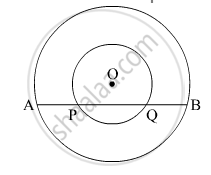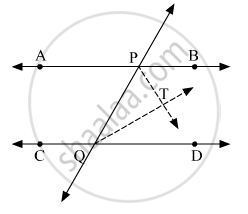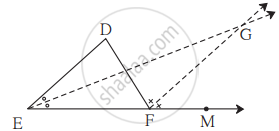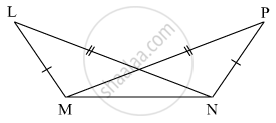# SSC (Marathi Semi-English) 9th Standard [इयत्ता ९ वी] - Maharashtra State Board Question Bank Solutions for Geometry

Subjects
Topics
Subjects
Popular subjects
Topics
Geometry
< prev  1 to 20 of 74  next >

In a parallelogram ABCD, If ∠A = (3x + 12)°, ∠B = (2x - 32)° then find the value of x and then find the measures of ∠C and ∠D.

Concept: Types of Quadrilaterals > Properties of a Parallelogram

In the given figure, centre of two circles is O. Chord AB of bigger circle intersects the smaller circle in points P and Q. Show that AP = BQ.[0.06] Circle
Chapter: [0.06] Circle
Concept: Properties of Chord

In the given Figure, line AB || line CD and line PQ is the transversal. Ray PT and ray QT are bisectors of ∠BPQ and ∠PQD respectively. Prove that m∠PTQ = 90°.[0.03] Triangles
Chapter: [0.03] Triangles
Concept: Remote Interior Angles of a Triangle Theorem

In the given figure, line DE || line GF, ray EG and ray FG are bisectors of ∠DEF and ∠DFM respectively. Prove that,
(i) ∠DEG = 1/2∠"EDF"
(ii) EF = FG.[0.03] Triangles
Chapter: [0.03] Triangles
Concept: Remote Interior Angles of a Triangle Theorem

In the following table, a ratio is given in each column. Find the remaining two ratios in the column and complete the table.

 sin θ 11/61 1/2 3/5 cos θ 35/37 1/sqrt3 tan θ 1 21/20 8/15 1/(2sqrt2)
[0.08] Trigonometry
Chapter: [0.08] Trigonometry
Concept: Trigonometric Ratios and Its Reciprocal
Write the following statement in conditional form.
Every rhombus is a square.
[0.01] Basic Concepts in Geometry
Chapter: [0.01] Basic Concepts in Geometry
Concept: Concept of Plane

In which quadrant are the following points ?

1.  whose both co-ordinates are positive.
2.   whose both co-ordinates are negative.
3.   whose x co-ordinate is positive, and the y co-ordinate is negative.
4.   whose x co-ordinate is negative and y co-ordinate is positive.
[0.07] Co-ordinate Geometry
Chapter: [0.07] Co-ordinate Geometry
Concept: Co-ordinates of Points on the Axes

As shown in the following figure, in ΔLMN and ΔPNM, LM = PN, LN = PM. Write the test which assures the congruence of the two triangles. Write their remaining congruent parts.[0.03] Triangles
Chapter: [0.03] Triangles
Concept: Congruence of Triangles

From the information given below, find which of the point is between the other two. If the points are not collinear, state so.

d(P, R) = 7,   d(P, Q) = 10,   d(Q, R) = 3

[0.01] Basic Concepts in Geometry
Chapter: [0.01] Basic Concepts in Geometry
Concept: Co-ordinates of Points and Distance

From the information given below, find which of the point is between the other two.
If the points are not collinear, state so.

d(R, S) = 8, d(S, T) = 6, d(R, T) = 4

[0.01] Basic Concepts in Geometry
Chapter: [0.01] Basic Concepts in Geometry
Concept: Co-ordinates of Points and Distance

From the information given below, find which of the point is between the other two.
If the points are not collinear, state so.

d(A, B) = 16,  d(C, A) = 9,   d(B, C) = 7

[0.01] Basic Concepts in Geometry
Chapter: [0.01] Basic Concepts in Geometry
Concept: Co-ordinates of Points and Distance

From the information given below, find which of the point is between the other two.
If the points are not collinear, state so.

d(D, E) = 5,   d(E, F) = 8,   d(D, F) = 6

[0.01] Basic Concepts in Geometry
Chapter: [0.01] Basic Concepts in Geometry
Concept: Co-ordinates of Points and Distance

From the information given below, find which of the point is between the other two.
If the points are not collinear, state so.

d(X, Y) = 15,   d(Y, Z) = 7,   d(X, Z) = 8

[0.01] Basic Concepts in Geometry
Chapter: [0.01] Basic Concepts in Geometry
Concept: Co-ordinates of Points and Distance

From the information given below, find which of the point is between the other two.
If the points are not collinear, state so.

d(L, M) = 11,   d(M, N) = 12,   d(N, L) = 8

[0.01] Basic Concepts in Geometry
Chapter: [0.01] Basic Concepts in Geometry
Concept: Co-ordinates of Points and Distance

Points X, Y, Z are collinear such that d(X,Y) = 17, d(Y,Z) = 8, find d(X,Z) .

[0.01] Basic Concepts in Geometry
Chapter: [0.01] Basic Concepts in Geometry
Concept: Co-ordinates of Points and Distance
Sketch proper figure and write the answer of the following question.
If A−B−C and l(AC) = 11, l(BC) = 6.5, then l(AB) = ?
[0.01] Basic Concepts in Geometry
Chapter: [0.01] Basic Concepts in Geometry
Concept: Co-ordinates of Points and Distance
Sketch proper figure and write the answer of the following question.
If R - S - T   and   l(ST) = 3.7,   l(RS) = 2.5,   then l(RT) =?
[0.01] Basic Concepts in Geometry
Chapter: [0.01] Basic Concepts in Geometry
Concept: Co-ordinates of Points and Distance
Sketch proper figure and write the answer of the following question.
If X - Y - Z  and   l(XZ) = 3√7, l(XY) = √7,  then l(YZ) =?
[0.01] Basic Concepts in Geometry
Chapter: [0.01] Basic Concepts in Geometry
Concept: Co-ordinates of Points and Distance
Select the correct alternative from the answer of the question given below.
Find d(A, B), if co-ordinates of A and B are -2 and 5 respectively.
[0.01] Basic Concepts in Geometry
Chapter: [0.01] Basic Concepts in Geometry
Concept: Co-ordinates of Points and Distance
Select the correct alternative from the answer of the question given below.
If P - Q - R and d(P,Q) = 2, d(P,R) = 10, then find d(Q,R)
[0.01] Basic Concepts in Geometry
Chapter: [0.01] Basic Concepts in Geometry
Concept: Co-ordinates of Points and Distance
< prev  1 to 20 of 74  next >